##### Calculus II Workbook For Dummies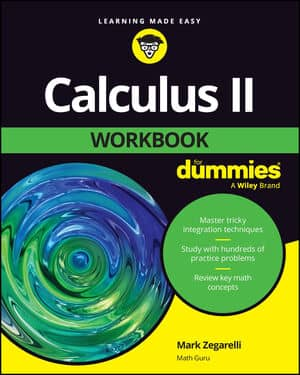Integrating by parts is the integration version of the product rule for differentiation. The basic idea of integration by parts is to transform an integral you can’t do into a simple product minus an integral you can do. Here’s the formula: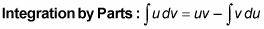Don’t try to understand this yet. Wait for the examples that follow.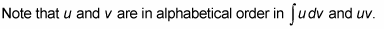If you remember that, you can easily remember that the integral on the right is just like the one on the left, except with the u and v reversed.

Here’s the method in a nutshell.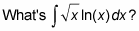First, you’ve got to split up the integrand into a u and a dv so that it fits the formula. For this problem, choose ln(x) to be your u. Then, everything else is the dv, namely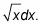Next, you differentiate u to get your du, and you integrate dv to get your v. Finally, you plug everything into the formula and you’re home free.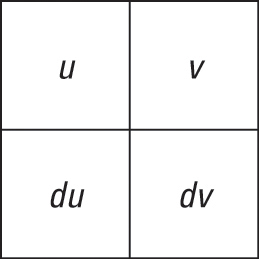The integration by parts box.
To help keep everything straight, organize integration-by-parts problems with a box like the one in the above figure. Draw an empty 2-by-2 box, then put your u, ln(x), in the upper-left corner and your dv,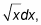in the lower-right corner, as in the following figure.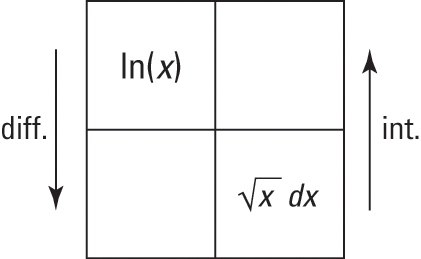Filling in the box.
The arrows in this figure remind you to differentiate on the left and to integrate on the right. Think of differentiation — the easier thing — as going down (like going downhill), and integration — the harder thing — as going up (like going uphill).

Now complete the box: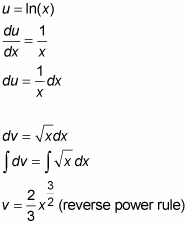The completed box for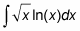is shown in the next figure.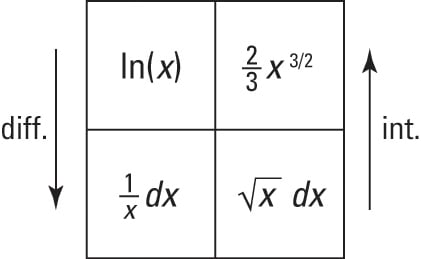A good way to remember the integration-by-parts formula is to start at the upper-left square and draw an imaginary number 7 — across, then down to the left, as shown in the following figure. This is an oh-so-sevenly mnemonic device (get it?—“sevenly” like “heavenly”—ha, ha, ha, ha.)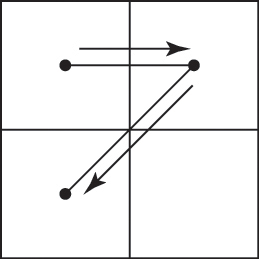Remembering how you draw the 7, look back to the figure with the completed box. The integration-by-parts formula tells you to do the top part of the 7, namely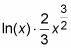minus the integral of the diagonal part of the 7,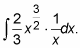(By the way, this method is much easier to do than to explain. Try the box technique with the 7 mnemonic. You’ll see how this scheme helps you learn the formula and organize these problems.)

Ready to finish? Plug everything into the formula: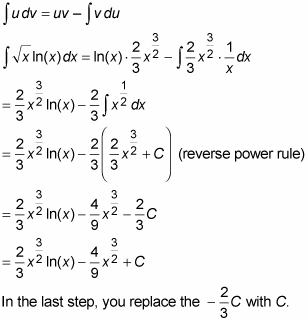This is because –2/3 times any number is still just any number.

Here’s a great mnemonic device for how to choose the u (again, once you’ve selected your u, everything else is automatically the dv.

Herbert E. Kasube came up with the acronym LIATE to help you choose your u (calculus nerds can check out Herb’s article in the American Mathematical Monthly 90, 1983 issue):
 L Logarithmic like log(x) I Inverse trigonometric like arctan(x) A Algebraic like 5x + 3 (x can be raised to any power) T Trigonometric like cos(x) E Exponential like 10 raised to the x power
(note that to qualify as an exponential function, the power must contain an x, not just a number.)